### Excel Financial Functions

Excel has a powerful pack of financial functions that you can use. Most of them are only used by people with specific needs, but some can be useful for the majority of people to make calculations on loans for instance. One of the most useful functions is PMT(). It can be used to calculate the monthly payment on a loan. Here’s the syntax:

PMT(rate, nper, pv, fv, type)

rate is the interest rate for the loan

nper is the number of payments for the loan

pv is the present value, is the loan amount

fv is an optional value. It can be the final payment for the loan. If omitted, it will assume 0

type can be either 0 or 1. It indicates when the payments are due. Default value is 0 assuming that the first payment is due after a month or you can set this to 1 if the first payment is to be done when the loan is issued.

Let see a practical example how we can use this function to calculate the monthly payment on a \$30,000 loan, with 5% annual interest rate and 72 monthly payments.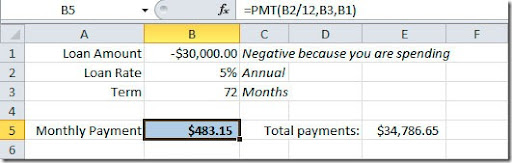As you can see on the image, on the formula bar, we have our annual rate divided by 12 because we want the monthly payment. The loan value need to be negative because this is a value that we are going to take “out of the bank”, we are spending it. This formula will result on a \$483.15 monthly payment witch will give us a total payment of \$34,786.65 over the 6 year period of the loan. Don’t forget that you have to add taxes and insurances values to this monthly payment.

We can use another version of our formula. Imagine that we want to leave \$10,000 for the end of the loan period. We can put this value on the pv argument of our formula and we will have this result: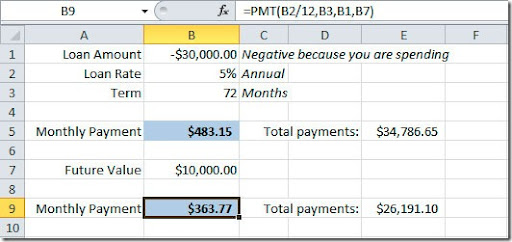If you check the formula on the formula bar, you will see that I’ve added the B7 cell as fv argument. So now we have a \$363.77 monthly payment with a final value of \$10,000.

No let’s do another kind of exercise. We know that we are making a monthly payment of \$500 on a \$30,000 loan amount and for 72 months. We want to figure out what is our annual interest rate. For that we need to use the RATE() function. Here’s the syntax:

RATE(nper, pmt, pv, fv, type, guess)

pv is the present value, is the loan amount

guess is your guess of what the rate will be. If omitted, it will assume that the rate is 10%.

Here’s how example would look like: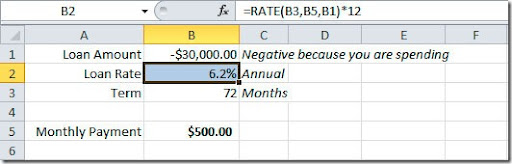Again, if you check our formula, we are multiplying the rate value for 12 months to get the annual rate. This formula will give us an annual interest rate of 6,2%.

But if we needed to calculate how much we could buy with a \$500 monthly payment over a 72 months period at a 5% interest rate? We need to calculate our “present value” (pv), right? For that we have the PV() function:

PV(rate, nper, pmt, fv, type)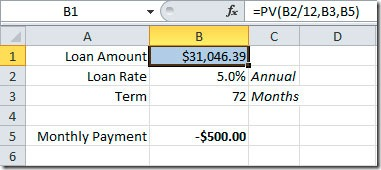As you can see, we could afford to spend as much as \$31,046.39 with those conditions.

Another useful financial function is FV(). It can be used to calculate a future value of an investment. Here’s the syntax:

FV(rate, nper, pmt, pv, type)

pv is the present value, or the money that you already have on the investment

Here’s an example of a retirement plan for a person that is 30 years now, is planning to retire at 65. This will give us a total of 420 monthly payments (35 years * 12 months). He plans to save \$100 a month and he will have a 3% annual interest rate on this financial product. He started with a \$5,000 deposit.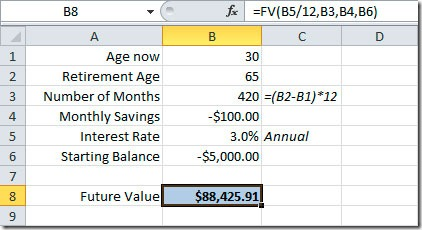As you can see, he will have, when he will retire at 65 years of age, \$88,425.91 on he’s savings account. If he started without making a deposit, you could omit the pv argument of the function.

Excel has much more financial functions but most of them are aimed to professionals that need to calculate investments analysis, depreciation calculations, etc, they are not aimed for the general Excel user. Maybe later we can see in detail some of them and how you can use them. For now, I hope that you find this basic financial functions useful.

### LARGE() Function–Get the top N values from a list

This is a real situation that many of us already experienced! We have a list of data and we need to get the top N values from that list. Of course we can sort descending the list and get the top values, but many times we can’t sort the source data (it may be used on another report, for instance). We can use the LARGE() function to get the nth largest value from a range of values. The syntax is like this:
LARGE(array, k)
array is the range of data from where we want to get our top values
k is the position on the range of data to return
So, let’s see an example on how to apply this function to get our top values. Our source data is like this:
In this example we want to get the Top 5 companies by Invoices value. We will be putting the values on cells B17:C21.
To start, let’s put the numbers 1 to 5 on cells A17:A21. Now, on cell C17 let’s put our formula, like this:
=LARGE(\$C\$3:\$C\$15, A17)
We will be looking on cells C3:C15, where we have our Invoices values, for the first largest value, that why we are using cell A17 that has the number 1 on it. Use the dollar signs on the array argument so that when we copy the formula to the next rows it will keep the reference to our Invoices values that are on C3:C15. Now copy the formula on cell C17 to the rest of the cells (C18:C21). This is how our sheet should look like:
So, now we have the top 5 Invoices values but wee need to fill the companies names. For that we will use the MATCH() function to get the row number within range C3:C15 that has the value that matches C17. The MATCH formula will be like this:
=MATCH(C17,\$C\$3:\$C\$15,0)
For the value in C17, this formula would return 10 because the value on this cell is coming from row 10 of our range source. This is not row 10 of our sheet but row 10 of our source! Now with this information we can get the company name that is on this row. For that we will use the INDEX() function like this:
=INDEX(\$B\$3:\$ B\$15,10)
This would get the company’s name on row 10 of our source data.
Combining both formulas, it will look like this:
=INDEX(\$B\$3:\$B\$15,MATCH(C17,\$C\$3:\$C\$15,0))
Put this formula on cell B17 and copy to the other cells on B18:B21.
The result will be like this, where we get on cells B17:C21 the top 5 values of Invoices from our source data on B3:C15:
Hope that this article can be useful for your work.

### SUMIF() and SUMIFS() Functions

To get the sum of values in a range of cells, we can use the SUMIF() function. The syntax is like this:

SUMIF(range, criteria, sum_range)

In this example we want to get the sum of sales from a region (“North”):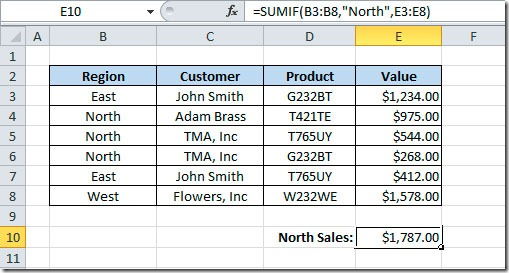As you can see on the formula bar, on cell E10 we have the following formula:

=SUMIF(B3:B8,”North”,E3:E8)

This will check on cells B3:B8 for the North region and will sum the values that correspond on cells E3:E8.

But if we wanted to get the sum of the North region for only a customer? Before Excel 2007, we needed to use the SUMPRODUCT() function. Now we have a new function in Excel that is called SUMIFS(). With this new function, we can have 2 or more conditions. Here’s the syntax:

SUMIFS(sum_range, criteria_range1, criteria1 [,criteria_range2, criteria2…])

The first argument is the range of cells to be summed. Then you should specify pairs of arguments that consist on the range to be check and the value to be match on that range. So, here’s an example of the sum of sales for the North region for customer “TMA, Inc”: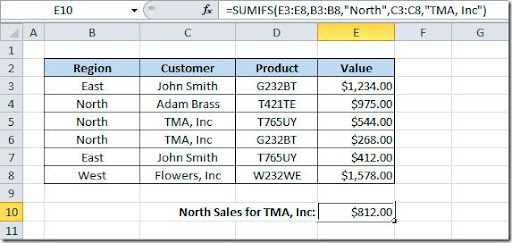If you check the formula bar, our formula will sum the values on cells E3:E8 that have the region North on cells B3:B8 and the customer “TMA, Inc” on cells C3:C8.

When moving from SUMIF to SUMIFS, you should pay attention because the syntax is in a different order.

### Cascading Validation Lists

Here’s the scenario: on our worksheet, we want to choose a Country and the corresponding City. We want to limit the countries available to the user as well as the cities that they can choose. For start, we want to choose the country and on the second cell we can only put cities that correspond to that country. It makes sense, right?
So, let’s start we our simple example on how we can make cascading (or dependent) Validation Lists. In cell A2 we want to put the country and on cell B2 we want to put the city, like this example: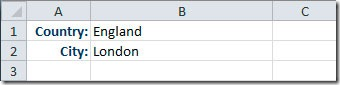To start, we have to build our “database”. On a second sheet, I’ve made a list of countries that I want to have available for the user to choose on my validation list and I putted and index number that will correspond to the right cities list. So, for instance, for France, I used the index 2 that will be corresponding to the cities list 2. You will see after how this index number will be used.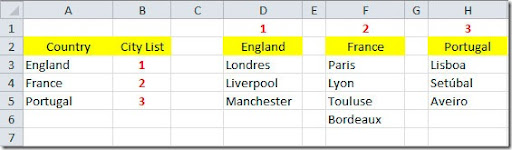Now, if you’re using Excel 2010, you can have the “source” lists on a separated sheet but if you’re using Excel 2003 for instance, you can only have the values on a second sheet if you use named ranges. We will use the name ranges so that this cascading validation lists work on both versions. So, the next step is to create the named ranges for our lists. On your Formulas tab, we have the Name Manager button. When you click it, it will open the Name Manager dialog box.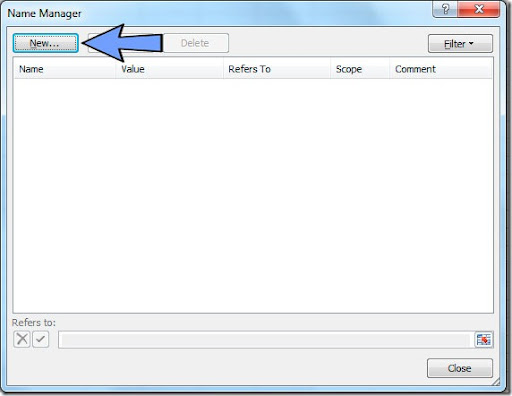Click on the New button to add a new named range and it will open the New Name dialog box.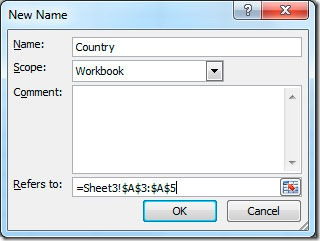On the Name field you can put, for instance, “Country” for our first named range and on the “Refers to” field we will select, in this case, =Sheet3!\$A\$3:\$A\$5 to get the list of countries. Click OK to finish. Then we need to create the named ranges for the rest of the lists. Click again on the New button on the Name Manager dialog box and repeat the process to create three more named ranges: England, France and Portugal, selecting for the “Refers to” field the corresponding range of cities names. This will leaves with 4 named ranges, like this: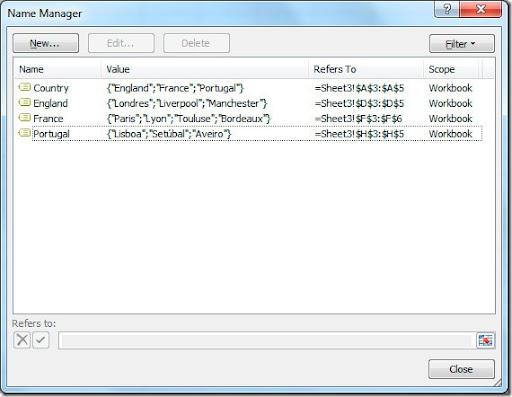Now we can build our validations lists on our original sheet where we want to select the data from our “database”. With cell B1 selected (where we want to select our country), go to the Data tab and click on Data Validation and choose Data Validation.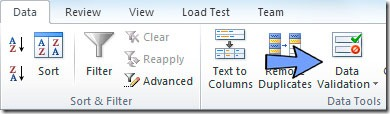This will open the Data Validation dialog box.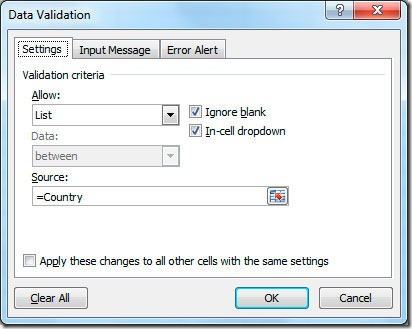Now we want to use a list of options for the user to select so go ahead and on “Allow” change the value to “List”. This will open the Source field that is where we will put our Country named range. So put =Country and click OK. Now we want to make the second Validation List for the user to choose the city. Select, in this case, cell B2 that is where we will put this second validation list. Now comes the trick. We need to check the value of the country cell (cell B1) to get the index of the corresponding value on our “database”. If the user selected Portugal we need to get the index “3” that will tell us to get the list of cities that correspond to Portugal. For that we will make a VLOOKUP of the value in cell B1 in the Countries table. After we get the index value, we will “choose” the list to use for the Source of our second validation list. To make this we will use this formula:

=CHOOSE(VLOOKUP(B1,Sheet3!\$A\$3:\$B\$5,2,FALSE),England,France,Portugal)

So, what does this formula do? It will start for looking up the value on cell B1 against the values on our countries table and getting the corresponding index that it will use on the CHOOSE() function to get either the 1st, 2nd or 3rd named range.

This is how our second validation list will look: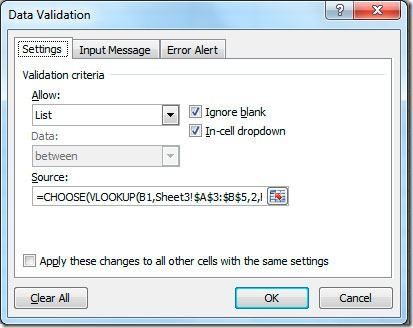The result will be two cascading or dependent Validation Lists. If we choose country France, we will have on the cities Validation List the cities from France only.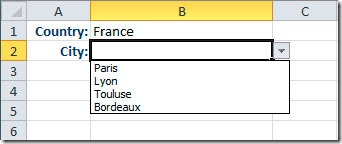This article demonstrates how you can make basic cascading Validation Lists to use on your sheets. You can take this example and use it on your own real life situations.